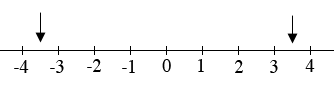SEARCH HOMEMath Central Quandaries & QueriesQuestion from priyanshi: Five rational numbers between -4 and -3Hi,

Look at the number lineWhat is the number half way from 3 to 4? What is the number half way between -3 and -4? What is the number half way between the number you just found and -3?

PennyMath Central is supported by the University of Regina and The Pacific Institute for the Mathematical Sciences.